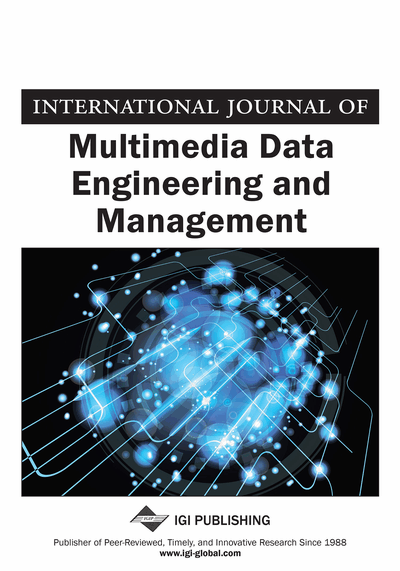# Implement Multichannel Fractional Sample Rate Convertor using Genetic Algorithm

Vivek Jain (The College of Technology and Engineering, Department of Electronics and Communication, Udaipur, India) and Navneet Agrawal (The College of Technology and Engineering, Department of Electronics and Communication, Udaipur, India)
DOI: 10.4018/IJMDEM.2017040102

## Abstract

In this paper reduce power of multichannel fractional sample rate convertor by minimized hamming distance between consecutive coefficients of filter using Genetic algorithm. The main component of multichannel fractional sample rate convertor is Cascaded multiple architecture finite impulse response filter (CMFIR filter). CMFIR is implemented by cascading of cascaded integrator-comb (CIC) & multiply accumulate architecture (MAC) FIR filter. Genetic algorithm minimizes the hamming distance between consecutive coefficients of CMFIR filter. By Minimizing the hamming distance of consecutive filter coefficient reduces the transaction from 0 to 1 or 1 to 0. These techniques reduce the switching activity of CMOS transistor which is directly reduces Dynamic power consumption by multichannel sample rate convertor, it also minimizes the total power consumption of multichannel fractional sample rate convertor. later than use genetic algorithm on 1 to 128 channel Down sample rate convertor total power reduced by 3.44% to 61.56%, dynamic power reduced by 9.09% to 56.25% .1 to 128 channel Up sample rate convertor total power reduced by 2.81% to 45.42%, dynamic power reduced by 4.76% to 56%, 1 to 128 channel fractional sample rate convertor total power reduced by 1.44% to 17.17%, dynamic power reduced by 6.25% to 19.92%.
Article Preview
Top

## Literature Review

Generally conventional multichannel fractional sample rate convertor implemented by using direct form FIR filter structure. The difficulty with build multichannel fractional Sample Rate Convertor with direct form architecture is filter length linearly increases with the decimation & Interpolation rate. Therefore, resource Utilization increases; this in turn increases Power consumption. To overcome this problem cascaded integrator-comb (CIC) filter structure to implement multichannel sample rate convertor. CIC filters can professionally carry out either diminish the sample rate or boost the sample rate, with two complementary structures integrator and comb filter being in use to execute these functions. The difficulty with execution of multichannel sample rate convertor with CIC filter was that, CIC filter was provide only for small bandwidth signals because mathematically the frequency response of CIC filter is given by a sinc function. The sinc function confined the bandwidth of multichannel fractional sample rate convertor in the main lobe width of sinc function. Our purposed model Cascaded multiple architecture finite impulse response (CMFIR) filter based multichannel fractional sample rate convertor is an efficient solution of that problem. CMFIR is implemented by cascading of CIC & multiply accumulate architecture (MAC) FIR filter. MAC architecture neutralizes the effect of sinc function of CIC filter. Now we apply genetic algorithm on coefficient of CMFIR filter to diminish the power consumption of multichannel fractional sample rate convertor by reducing the hamming distance of consecutive coefficient of CMFIR filter. The basic component of CMFIR filter is CMOS transistor when it is implemented on FPGA. By reducing the hamming distance of consecutive coefficient of CMFIR filter reduces the switching of CMOS transistor for transition from 0 to 1 or 1 to 0 without effecting frequency response of multichannel fractional sample rate convertor, where:= Total power consumption by CMOS transistor= Static power consumption by CMOS transistor= Dynamic power consumption by CMOS transistor= Transient power consumption= Capacitive load power consumption= Dynamic power dissipation in capacitance= Number of bits toggling from 0 to 1 or 1 to 0 of consecutive CMFIR filter coefficient= Input signal frequency= Output signal frequency

The total power consumption by CMOS transistor is given by:

(1)(2)(3)(4)

## Complete Article List

Search this Journal:
Reset
Open Access Articles: Forthcoming
Volume 11: 4 Issues (2020): 1 Released, 3 Forthcoming
Volume 10: 4 Issues (2019)
Volume 9: 4 Issues (2018)
Volume 8: 4 Issues (2017)
Volume 7: 4 Issues (2016)
Volume 6: 4 Issues (2015)
Volume 5: 4 Issues (2014)
Volume 4: 4 Issues (2013)
Volume 3: 4 Issues (2012)
Volume 2: 4 Issues (2011)
Volume 1: 4 Issues (2010)
View Complete Journal Contents Listing